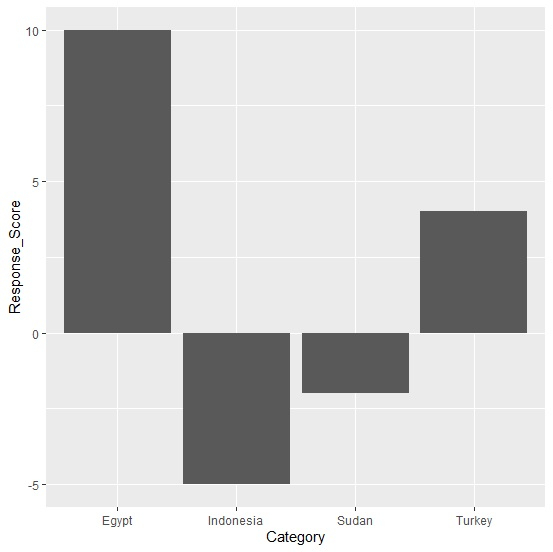# How to create bar plot with positive and negative values in R?

To create bar plot with positive and negative values, we can make use of ggplot function.

For example, if we have a data frame called df that contains a categorical column say C and a numerical column Num which has some positive and some negative values then the bar plot for this data can be created by using the below command −

ggplot(df,aes(C,Num))+geom_bar(stat="identity")

## Example

Following snippet creates a sample data frame −

Category<-c("Egypt","Sudan","Turkey","Indonesia")
Response_Score<-c(10,-2,4,-5)
df<-data.frame(Category,Response_Score)
df

## Output

The following dataframe is created −

  Category  Response_Score
1  Egypt      10
2  Sudan      -2
3  Turkey      4
4  Indonesia  -5

To load ggplot2 package and create bar chart for data stored in df, add the following code to the above snippet −

library(ggplot2)
ggplot(df,aes(Category,Response_Score))+geom_bar(stat="identity")

## Output

If you execute all the above given snippets as a single program, it generates the following output −